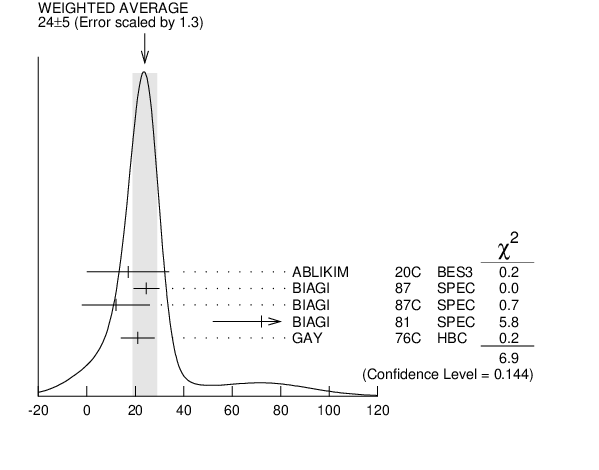# ${{\boldsymbol \Xi}{(1820)}}$ WIDTH INSPIRE search

VALUE (MeV) EVTS DOCUMENT ID TECN CHG  COMMENT
$\bf{ 24 {}^{+15}_{-10}}$ OUR ESTIMATE
$\bf{ 24 \pm6}$ OUR AVERAGE  Error includes scale factor of 1.5.
$24.6$ $\pm5.3$ 280 1
 1987
SPEC 0 ${{\mathit \Xi}^{-}}$ ${}^{}\mathrm {Be}$ $\rightarrow$ ( ${{\mathit \Lambda}}{{\mathit K}^{-}}$) X
$12$ $\pm14$ $\pm1.7$ 54
 1987 C
SPEC 0 ${{\mathit \Xi}^{-}}$ ${}^{}\mathrm {Be}$ $\rightarrow$ ( ${{\mathit \Lambda}}{{\overline{\mathit K}}^{0}}$) X
$72$ $\pm20$ 300
 1981
SPEC - SPS hyperon beam
$21$ $\pm7$ 130
 1976 C
HBC - ${{\mathit K}^{-}}{{\mathit p}}$ 4.2 ${\mathrm {GeV/}}\mathit c$
• • • We do not use the following data for averages, fits, limits, etc. • • •
$23$ $\pm13$
 1999 B
WA89 ${{\mathit \Sigma}^{-}}$ nucleus, 345 GeV
$99$ $\pm57$ 74
 1977
HBC 0 ${{\mathit K}^{-}}{{\mathit p}}$ 2.87 ${\mathrm {GeV/}}\mathit c$
$52$ $\pm34$ 68
 1977
HBC -0 ${{\mathit \Xi}{(1530)}}{{\mathit \pi}}$
$72$ $\pm17$ 39
 1977
HBC - ${{\mathit \Sigma}^{-}}{{\overline{\mathit K}}^{0}}$
$44$ $\pm11$ 44
 1977
HBC 0 ${{\mathit \Lambda}}{{\overline{\mathit K}}^{0}}$
$26$ $\pm11$ 57
 1977
HBC - ${{\mathit \Lambda}}{{\mathit K}^{-}}$
$85$ $\pm58$
 1975
DBC -0 ${{\mathit \Xi}}{{\mathit \pi}}{{\mathit \pi}}$ , ${{\mathit \Xi}^{*}}{{\mathit \pi}}$
$51$ $\pm13$ 2
 1972
HBC -0 Lower mass
$58$ $\pm13$ 2
 1972
HBC -0 Higher mass
$103$ ${}^{+38}_{-24}$ 3
 1970 B
DBC -0 3.6, 3.9 ${\mathrm {GeV/}}\mathit c$
$48$ ${}^{+36}_{-19}$ 4
 1970 B
DBC -0 3.6, 3.9 ${\mathrm {GeV/}}\mathit c$
$55$ ${}^{+40}_{-20}$
 1969
HBC - ${{\mathit \Lambda}}$ , ${{\mathit \Sigma}}{{\overline{\mathit K}}}$
$12$ $\pm4$
 1965
HBC 0 ${{\mathit \Lambda}}{{\overline{\mathit K}}^{0}}$
$30$ $\pm7$
 1965 B
HBC -0 ${{\mathit \Lambda}}{{\overline{\mathit K}}}$
$\text{<80}$
 1963
FBC -0 ${{\mathit K}^{-}}$ freon 3.5 ${\mathrm {GeV/}}\mathit c$
1  BIAGI 1987 also sees weak signals in the in the ${{\mathit \Xi}^{-}}{{\mathit \pi}^{+}}{{\mathit \pi}^{-}}$ channel at $1782.6$ $\pm1.4$ MeV ($\Gamma$ = $6.0$ $\pm1.5$ MeV) and $1831.9$ $\pm2.8$ MeV ($\Gamma$ = $9.6$ $\pm9.9$ MeV).
2  BADIER 1972 adds all channels and divides the peak into lower and higher mass regions. The data can also be fitted with a single Breit-Wigner of mass 1800 MeV and width 150 MeV.
3  From a fit to inclusive ${{\mathit \Xi}}{{\mathit \pi}}$ , ${{\mathit \Xi}}{{\mathit \pi}}{{\mathit \pi}}$ , and ${{\mathit \Lambda}}{{\mathit K}^{-}}$ spectra.
4  From a fit to inclusive ${{\mathit \Xi}}{{\mathit \pi}}$ and ${{\mathit \Xi}}{{\mathit \pi}}{{\mathit \pi}}$ spectra only.${{\mathit \Xi}{(1820)}}$ width (MeV)
References:
EPJ C11 271 Production of ${{\mathit \Xi}^{*}}$ Resonances in ${{\mathit \Sigma}^{-}}$ Induced Reactions at 345 ${\mathrm {GeV/}}\mathit c$
 BIAGI 1987
ZPHY C34 15 ${{\mathit \Xi}^{*}}$ Resonances in ${{\mathit \Xi}^{-}}{}^{}\mathrm {Be}$ Interactions. 1. Diffractive Production in the ${{\mathit \Lambda}}{{\mathit K}^{-}}$ and ${{\mathit \Xi}^{-}}{{\mathit \pi}^{+}}{{\mathit \pi}^{-}}$ Channels
 BIAGI 1987C
ZPHY C34 175 ${{\mathit \Xi}^{*}}$ Resonances in ${{\mathit \Xi}^{-}}{}^{}\mathrm {Be}$ Interactions. 2. Properties of ${{\mathit \Xi}{(1820)}}$ and ${{\mathit \Xi}{(1950)}}$ in the ${{\mathit \Lambda}}{{\overline{\mathit K}}^{0}}$ and ${{\mathit \Sigma}^{0}}{{\overline{\mathit K}}^{0}}$ Channels
 BIAGI 1981
ZPHY C9 305 Production of Hyperons and Hyperon Resonances in ${{\mathit \Xi}^{-}}{{\mathit N}}$ Interactions at 102 and 135 ${\mathrm {GeV/}}\mathit c$
 BRIEFEL 1977
PR D16 2706 Search for ${{\mathit \Xi}^{*}}$ Production in ${{\mathit K}^{-}}{{\mathit p}}$ Interactions at 2.87 ${\mathrm {GeV/}}\mathit c$
 GAY 1976C
PL 62B 477 Production and Decay of ${{\mathit \Xi}^{*}{(1820)}}$ in ${{\mathit K}^{-}}{{\mathit p}}$ Reactions at 4.2 ${\mathrm {GeV/}}\mathit c$
 DIBIANCA 1975
NP B98 137 Study of ${{\mathit \Xi}^{-}}$ and ${{\mathit \Omega}^{-}}$ Production from ${{\mathit K}^{-}}{{\mathit n}}$ and ${{\mathit K}^{-}}{{\mathit p}}$ Interactions at 4.93 ${\mathrm {GeV/}}\mathit c$
NP B37 429 Search for ${{\mathit \Xi}^{*}}$ Resonances in ${{\mathit K}^{-}}{{\mathit p}}$ Interactions at 3.95 ${\mathrm {GeV/}}\mathit c$
PR D1 847 Study of ${{\mathit \Xi}^{*}}$ Resonances in ${{\mathit K}^{-}}{{\mathit n}}$ Interactions at 3.6 and 3.9 GeV
PL 16 171 Baryonic States of Strangeness $-2$ Produced in ${{\mathit K}^{-}}{{\mathit p}}$ Interactions at 3.0 ${\mathrm {GeV/}}\mathit c$